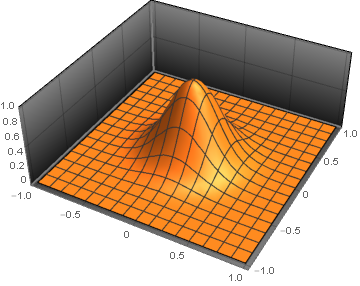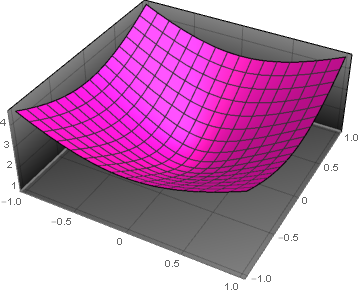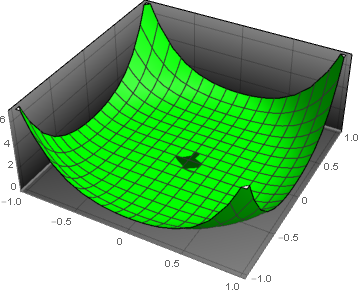Our Research Group

• Advisor : Dr.Cecile Piret - Department of Mathematical Sciences,MTU
• Professor John Gierke - Department of Geo-sciences, MTU
• Professor Bengt Fornberg - Department of Mathematics, University of Colorado Bolder
• Jacob Blazejewski - Graduate Student, Department of Mathematical Sciences, MTU

You download codes that I use in my research or other numerical analysis
codes from my GitHub account.

Applied Mathematics Research

My research focuses on using the Finite Difference-based Radial Basis Functions (RBF-FD) method to solve partial differential equations with applications to geology.

My first research project was about solving groundwater flow and contaminant transport equations in a heterogeneous geological environment. With this procedure, we combine RBF's strengths in representing complex geometries with its ability to accurately discretize differential operators, and attain a new robust modeling approach for stably and accurately computing the solution even in the vicinity of active wells, mathematically represented as singularities. We validated our method and verified its high order of accuracy by solving a well-known benchmark problem. Numerical results for groundwater flow equations in heterogeneous media were compared to results obtained with the USGS Finite Difference-based software MODFLOW that is customarily used by the geological community.

Current Projects

Our current project focuses on solving groundwater flow equations when there are fractures present on the domain. Often the fractures will be represented by discontinuous functions in the PDE.  When there are discontinuous coefficients present in the PDE, it is very hard to solve it numerically. We are working on a method that can handle these discontinuities just like we handled the singularities in the PDE in the previous project.

A real valued function, that only depends on the distance from the origin or distance from a center node is called a radial basis function. Here are three famously used RBFs.If you are interested in my research, watch the following video series to learn about our first project.

In Preparation

• The RBF-FD method for solving groundwater equations in the presence of fractures. Dissanayake N., Piret C.,  Gierke J.S., Fornberg B.
• Parareal--RBF--FD Methods for solving Time-Dependent PDEs. Dissanayake N., Ong B., Piret C..
• RBFs for solving Maxwell’s equation in the context of modeling phenomena in optics and photonics. El - Ganainy R., Mahalleh M., Piret, C., Dissanayake, N.

Part 2

Contact Information

• Office: Fisher 327A